# Static Members in C++

## Static Members in C++ with Examples:

In this article, I am going to discuss Static Members in C++ with Examples. Please read our previous article where we discussed Friend Function and Friend Classes in C++ with Example. The static is a keyword in the C++ programming language. We use the static keyword to define the static data member or static member function inside and outside of the class.

##### Static Data Members in C++

We can define class members’ static using the static keyword in C++. When we declare a member of a class as static it means no matter how many objects of the class are created, there is only one copy of the static member available throughout the program. Let us understand Static Members in C++ with some examples. For explaining static members, we have taken an example here.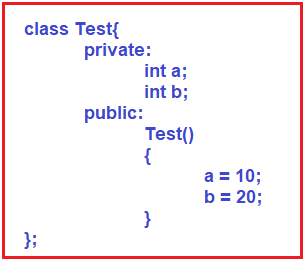This is our class Test. This class is having two private members that are a and b and it is having one public constructor that is for initializing a and b. So, it is a simple class that has two private members and a constructor for initializing those private members. Then inside the main function, we have created two objects of class Test as follows: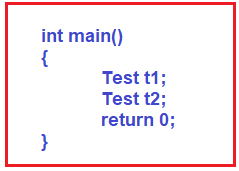Here we have created two objects t1 and t2 for the class Test. When we have created t1 then the constructor will be called t1 for and that will initialize a and b. And same holds for t2. For a better understanding, please have a look at the below image.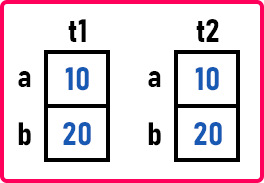So, we have created two objects for the Test class. Then every object will have it’s own a and b data members. Now let us understand static data members. We are defining a static variable in the above class as follows: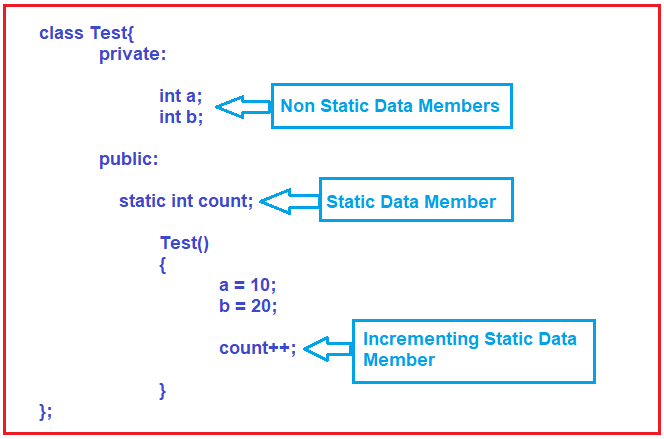So here inside the Test class, we have declared a static integer type variable that is count. And also, we are incrementing its value by 1 in the constructor of the class Test. Here, the count is a static variable. Then how does it works? When we are creating an object t1 then how many members are there and for which memory will be allocated? a, b and count. And when we are creating an object t2, again a, b, and the count will be there. For a better understanding, please have a look at the below image.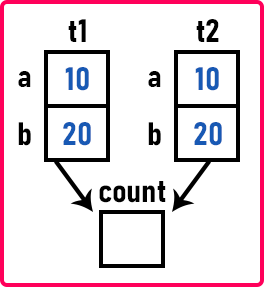As you can see in the above diagram, both t1 and t2 have separate memory allocations for their a and b data members but the same memory is shared for the count variable.

So how many times this count variable is allocated? Only one time the memory is allocated. And that memory is shared by both the objects t1 and t2. Both of them will use the same count. So, it means when we make a variable as static, that variable will occupy memory only once. So static variables or static data members of a class belong to a class. That doesn’t belong to an object. And all the objects can share it. So, there will be only one copy and every object will share that copy.

Now let us see what happens. At the time of creating t1, the constructor will assign a and b variables to 10 and 20 and increment the count variable by 1. Assume the initial value of the count is 0. So, after creating the object t1, the count will become 1 as shown in the below image.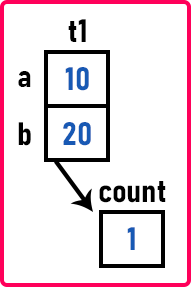Then when we were creating t2, the constructor was called that assign t2’s a and b to 10 and 20 and increments the count. So, previous the count value was 1, then after incrementing, it will become 2 as shown in the below image.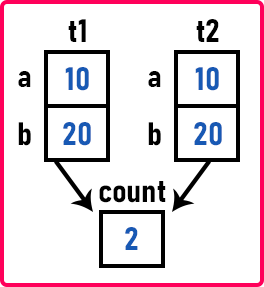So, both t1 and t2 have to access the same count variable. Such data members are static data members of a class. Static members are belonging to a class and they are common to all the objects. So static members are shareable members of a class.

One more thing we have to show you syntactically. When you have a static variable inside the class, you must have declared it outside again. So, when we declare the class variable outside the class, then it will become a global variable but we want it to be accessible only inside the Test class. So, we can use the scope resolution operator to make it accessible only inside the Test class. The statement is,

int Test::count = 0;

This is an important statement. When you declared a static member inside a class then that data member must be declared outside the class again using the scope resolution operator.

Now count is a global variable. A global variable is common for the whole program. There is only one variable created for the entire program. But we want the count variable to be used only within the Test class. So, for this, we have given scope resolution to count. So, two times we need the declaration of the count variable. It is just like a global variable and you are making it accessible only to the objects of the Test class. So that is the static variable. Only one-time memory is allocated and all objects can access it. That’s all about static data members.

##### How to access Static Data Members in C++?

Now let us see how to access that static variable.
cout << t1.count << endl;

Can we access it like this? Yes, we can access it because it is public inside the class. So, what will be displayed here? It will display 2.
cout << t2.count << endl;

Can we say this? Yes. So, what will be displayed here? Again 2 will be displayed. As count is public, so we can also write,
cout << Test::count << endl;

Again 2 will be displayed. So here we have learned a new thing. Static data members can be accessed using objects or they can also be accessed using the class name if they are public. You can directly access them using the class name. So static member actually belongs to a class and hence we can access them using the class name.

##### Example to Understand Static Data Members in C++:
```#include <iostream>
using namespace std;
class Test
{
private:
int a;
int b;
public:
static int count;
Test()
{
a = 10;
b = 20;
count++;
}
};

int Test::count = 0;

int main()
{
Test t1;
Test t2;
cout << t1.count << endl;
cout << t2.count << endl;
cout << Test::count << endl;
return 0;
}
```
###### Output: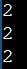Note: When we define the data member of a class using the static keyword, the data members are called the static data member.

##### Static Member Functions in C++:

Now we will discuss static member functions in C++. By declaring a member function as static in C++, we make it independent of any particular object of the class. A static member function can be called even if no objects of the class exist and the static functions are accessed using the class name and the scope resolution operator (::) and even we can also access them using objects of the class. Let us see. We are writing a static member function as follows: We have written this function inside our Test class.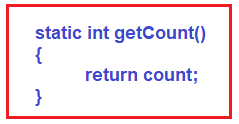This is a Static Member Function in C++ which is returning the value of the static count variable. Now let us understand something. Inside getCount function, can we write a++? No, it’s not allowed. But can we say count++ or return count? Yes, we can access count but you cannot access a or b. So, the important thing is Static Member Functions in C++ can access only static data members of a class. They cannot access non-static data members. So static member functions also belong to a class. Let us see.

cout << Test::getCount() <<endl;

Can we call the getCount function? Yes, we can call it. We can call it by using the scope resolution operator. This function will return the value of the count then that value will be printed on the screen. So, what is the count initially? We did not create any object so the count is never incremented. So, the value of the count is zero. Now if we write,

Test t1;
cout << t1.getCount() << endl;

Can we call this function getCount upon an object of class Test? Yes. It is just like static variables. You can access static functions also by using the class name as well as using an object. Now let us look at the program.

##### Example to Understand Static Members in C++:
```#include <iostream>
using namespace std;

class Test
{
public:
int a;
static int count;

Test()
{
a = 10;
count++;
}

static int getCount()
{
return count;
}

};

int Test::count = 0;

int main()
{
cout << "Calling count without object: " << Test::count << endl;
cout << "Calling getCount without object: " << Test::getCount() << endl;
Test t1;
cout << "Calling count with object t1: " << t1.count << endl;
cout << "Calling getCount with object t1: " << t1.getCount() << endl;
}
```
###### Output: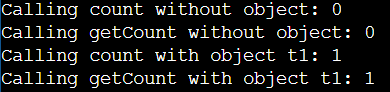Note: The static member functions are special functions used to access the static data members or other static member functions.

##### Key Points on Static Members in C++:
1. Static data members are members of a class.
2. Only one instance of static members is created for the entire class and shared by all objects of that class, no matter how many objects are created.
3. It is initialized before any object of this class is created, even before the main starts.
4. They can be accessed directly using the class name.
5. Static member functions are functions of a class, they can be called using the class name, without creating the object of a class.
6. They can access only static data members of a class; they cannot access non-static members of a class.
7. It is visible only within the class, but its lifetime is the entire program.